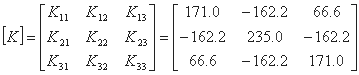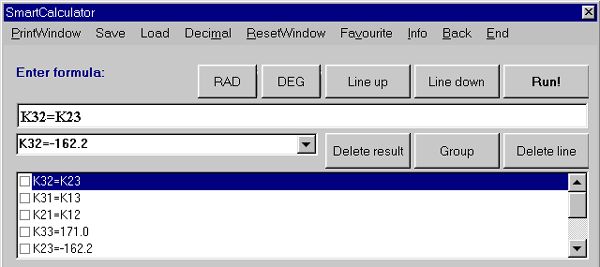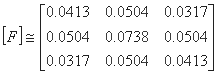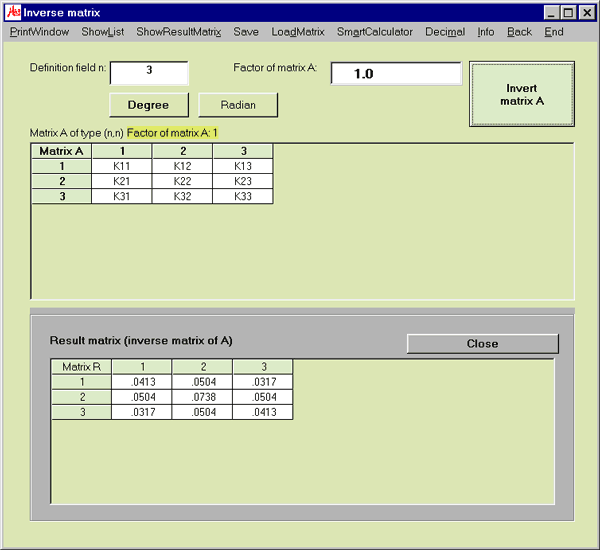# MasterAllRound: the PC calculator

## Matrices: example inverse matrix: flexibility matrix

This example is from the area of finite element analysis. The task is to get the flexibility matrix [F]. The flexibility matrix is the inverse matrix of the stiffness matrix [K]. The example shows only a 3x3 matrix but there is no size restriction for MasterAllRound.

Let's assume the following stiffness matrix:To solve this problem, more than one input method exists in MasterAllRound. We will show you the solution using number symbols (constants).
• Using the smart calculator of MasterAllRound, we declare the values of the number symbols K11, K12 et cetera first:  K11 = 171.0 and K12 = -162.2 and K13 = 66.6 K21 = K12 and K22 = 235.0 and K23 = -162.2 K31 = K13 and K32 = K23 and K33 = 171.0• After this, we enter the size of the matrix (n=3)
• We will represent the cells with the number symbols K11, K12 et cetera
• We start the calculation of the inverse matrix by clicking on the "Invert matrix A" button

The result is:Here you see the MasterAllRound window with the input and the result matrix: